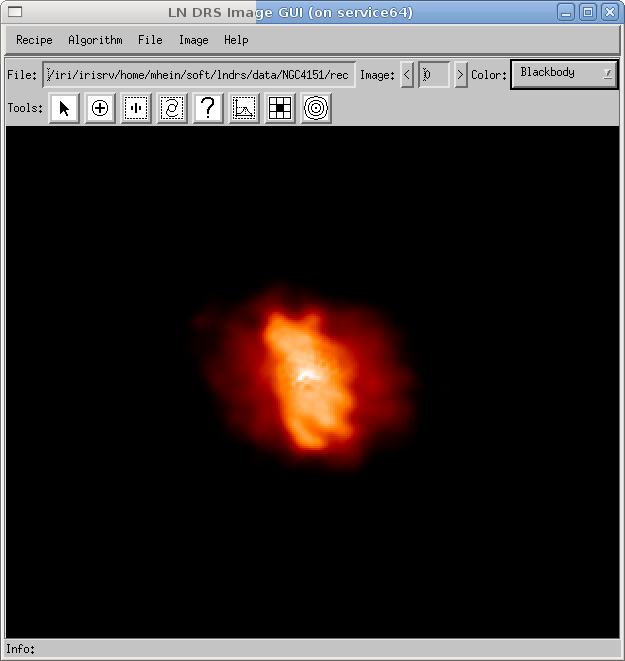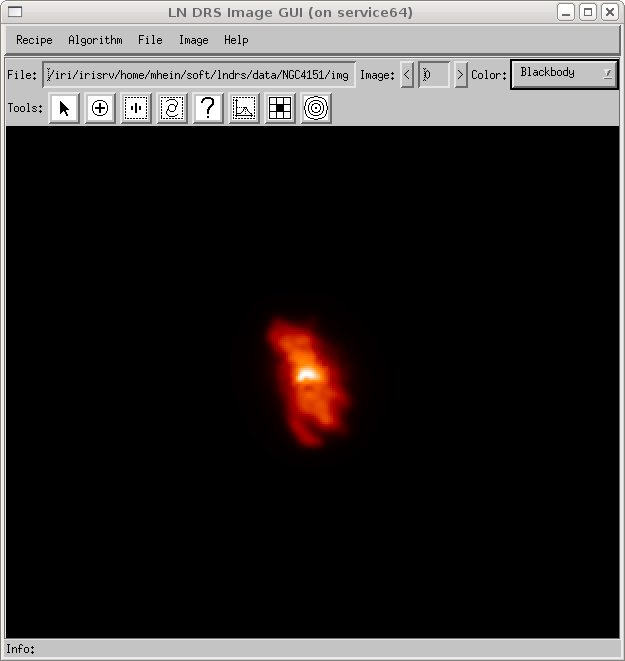# Introduction

Reconstructing high-resolution images from several target images at different position angles and associated psfs is the primary task of the LINC-NIRVANA Data Reduction Software (LN DRS). All previous steps provide the input for the last deconvolution step. The kind of target and psf images depends on the algorithm selected for deconvolution. Typical images used by the Richardson-Lucy (RL) algorithm are shown in figure 1.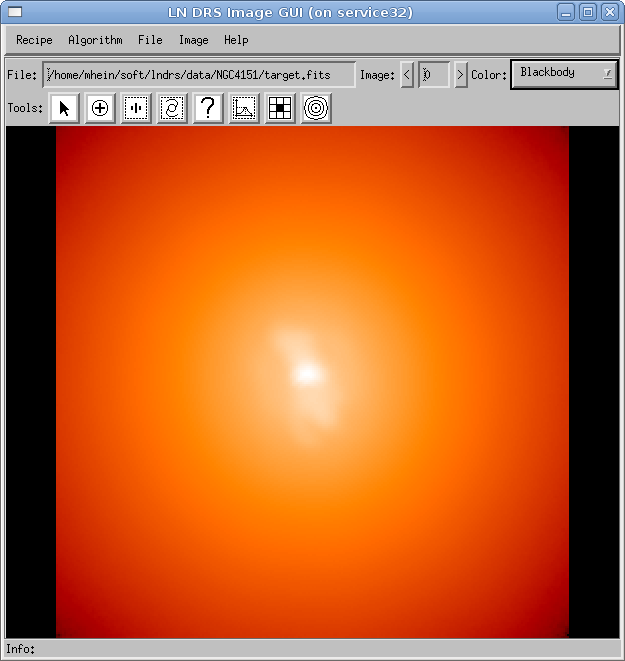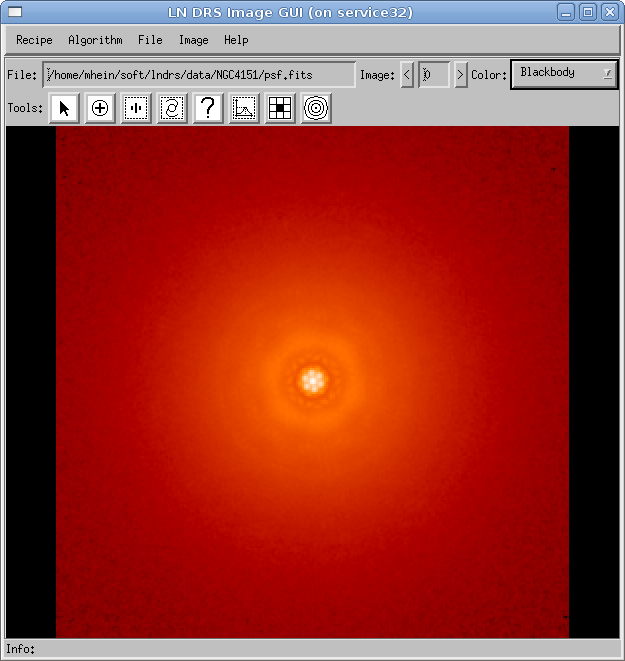The necessary parameters of each deconvolution algorithm can be set in the deconvolution algorithm dialog box and on the target and psf images. Further information is given in the reference section (see section Reconstruction algorithms). A typical dialog window is shown in figure 2 (Richardson-Lucy).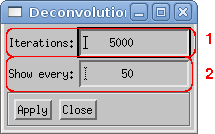In the dialog window, the following elements are shown:

1. The total number of iterations the algorithm should be use. The RL algorithm does not stop until this number of iterations is executed.
2. If the algorithm is written in IDL, this number means that the result from each specified iteration should be shown in the image window.

The execution of an algorithm written in IDL may be interrupted by using the menu item Algorithm/Pause or the Pause button below the algorithm list in the recipe GUI. With the menu item Algorithm/Resume or the Resume button below the algorithm list in the recipe GUI, the execution can be resumed. Figure 3 shows a reconstruction convolved with the ideal 23m psf in addition to the normal reconstruction.